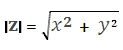# SQL Server IMABS function

Updated: 27 August 2010

Use wct.IMABS function to calculate the absolute value of a complex number in the x + yi or x + yj format. The absolute value of a complex number is calculated as:where:
z = x + yi
Syntax
SELECT [wctEngineering].[wct].[IMABS] (
<@inumber, nvarchar(4000),>)
Arguments
@inumber
is the value at which to evaluate the function. @inumber is an expression of type varchar or of a type that can be implicitly converted to varchar and must be in the format x + yi or x + yj, where x is a real number that can converted to float and yi (or yj) is an imaginary number, where the y part can be converted to float.
Return Types
float
Remarks
·         Use COMPLEX to convert real and imaginary coefficients into a complex number
·         IMABS(@inumber) = SQRT(power(IMREAL(@inumber), 2)+power(IMAGINARY(@inumber, 2)))
Examples

select
wct.IMABS('7+24i')

This produces the following result
----------------------
25

(1 row(s) affected)

select
SQRT(power(wct.IMREAL('7+24i'), 2)+power(wct.IMAGINARY('7+24i'), 2))

This produces the following result
----------------------
25

(1 row(s) affected)

### SupportCopyright 2008-2021 Westclintech LLC         Privacy Policy        Terms of Service